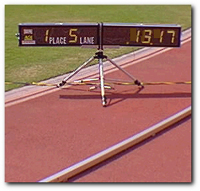The Daniels/Gilbert Formula--Page 2

 The first formula that I want to show you is called the Oxygen Cost Formula: O2cost = 0.182258 X (velocity) + 0.000104 x (velocity)2 - 4.60 The reference to "velocity" is, of course, referring to the running velocity. Like all scientific formula's, it uses the metric system. This means the velocity is expressed in meters per minute. The oxygen cost is expressed in milliliters of oxygen per kilogram of the runner's weight per minute. If you are an American, this requires a little math on your part because you are used to expressing your pace in terms of so many minutes per mile, and not meters per minute.So let's assume for illustration purposes you want to run the 5km distance in a time of 13:17. What is the oxygen cost? The first thing you have to do is convert that time to a velocity expressed in meters per minute. 13:17 is really 13.28 minutes. So if we divide 5000 meters by 13.28 minutes, we will find the number of minutes per meter we would be running in order to stay "on pace". That turns out to be around 376 meters per minute. (5000/13.28 = 376) If we now substitute that amount in our formula, we get: O2cost = 0.182258 X (376) + 0.000104 x (376)2 - 4.60 O2cost = 68.53 + 14.70 - 4.60 O2cost = 78.63 ml/kg/min This information probably doesn't mean much to you at the moment. You will see its significance later however when we combine this information with the Daniels/Gilbert Drop Dead Formula. .